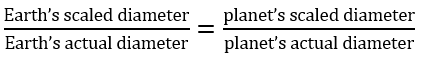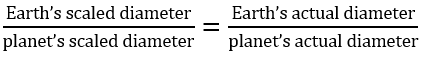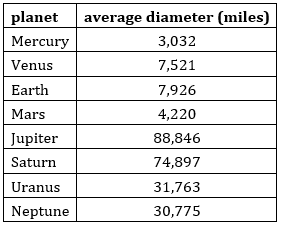# G.3.2.2 Shrinking the Solar System

In the opener we used proportional relationships to find the moon's scaled diameter when the earth was the size of a tennis ball - a diameter of 2.575 inches. Let's find out what the scaled diameter of all of the planets in our solar system would be. Use either of these equivalent ratio equations to calculate the scaled diameter for any of the planets below.The diameter of the sun is about 864,327 miles. What would the scaled diameter of the sun be if the earth was the size of a tennis ball - 2.575 feet?